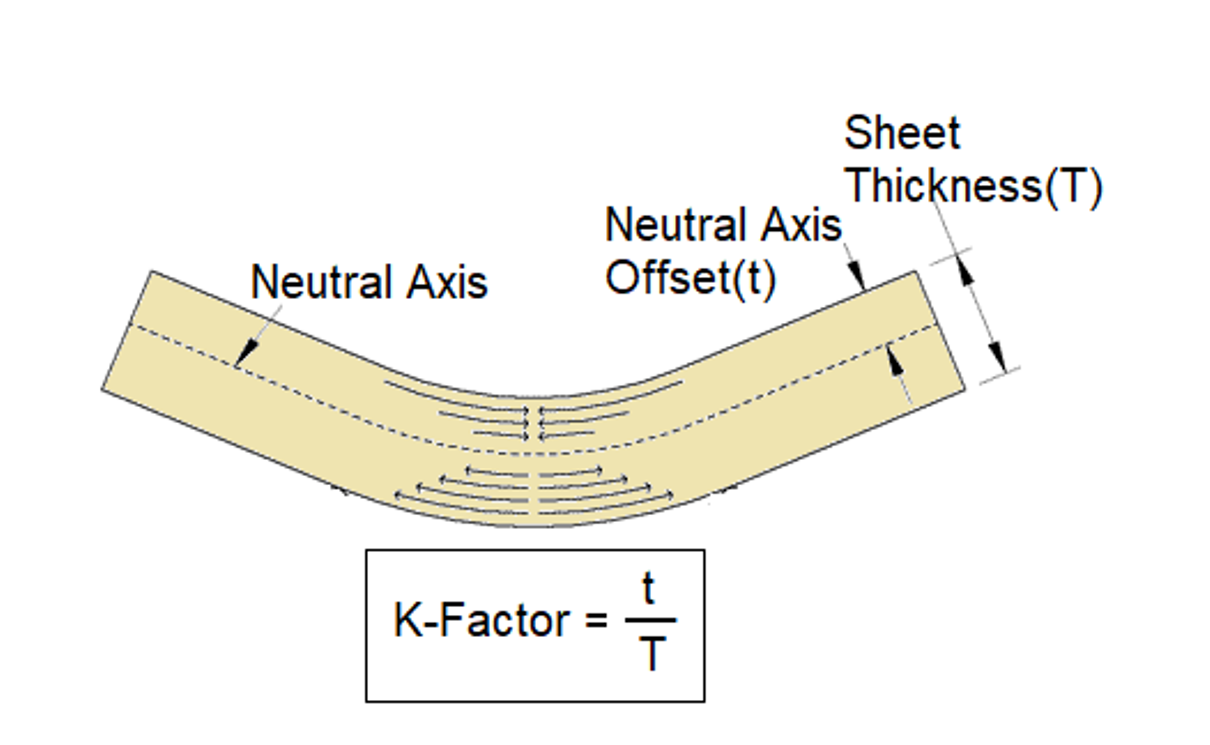# Sheet Metal K Factor (with Calculator and Formula)

In my previous post, I have talked about Bend Deduction and Bend Allowance. Adding more in this series of the terminology used in Sheet Metal Design, today I am going to write on the Sheet Metal K Factor.

Do you know when you bend a piece of sheet metal, one portion of its section undergoes compression and the other one undergoes tension. Compression occurs in the part of the section which besides the inside radius and the tension occurs in the part which besides the outside radius. There is a line in the section where neither compression nor tension takes place. This line is called the neutral axis. The transition from compression to tension takes place from this neutral axis.

The neutral axis is exactly in the middle of the section when the sheet is flat or unbent. But when you bend it, the neutral axis moves towards the inside bend. Hence this relocation of the neutral axis causes a change in its distance from the inside bend. But the length of the neutral axis remains the same even after the bend.

## What is the K Factor in Sheet Metal?

K-Factor is actually the ratio of the offset (t) of the neutral axis from the inside bend and the thickness of the sheet (T) when a piece of sheet metal is bent. As I have shown in the images below.Now, you may be thinking, where this k factor is used. Let me make it clear that it is a very important term used in sheet metal design. It is used to calculate Bend Allowance and Bend Deduction which are further used to calculate the flat pattern. Hence all of the sheet metal designing software use K-Factor, in order to provide an accurate design of the flat pattern of the sheet metal component.

The location of the neutral axis is not the same in all cases. Hence, the K-Factor is different for different cases. And it depends on some factors like:

• Material Thickness
• Material’s Physical Properties
• Sheet Metal Forming Method

Hence the value of the K-Factor is different for the different materials, inside bend radius, method of forming and different thickness. It ranges from 0 to 0.5. But practically, it is found to be between 0.3 and 0.5 in usual cases.

## K Factor Calculator

You can use a K Factor Chart to collect the value of K-Factor for the given case. But If you want to calculate it by yourself, you can make a trial on some sample pieces. You can try on 3-5 samples. Follow the following steps as you K Factor Calculator:

1. Prepare 5 sample blanks of the same size (same width and length)
2. Bend the sample pieces by using the tooling which you are going to use in the actual forming of the final pieces.
3. Now, accurately measure the flange lengths of all of the samples of the sheet metal pieces. Take an average dimension out of them.
4. Also, take accurate measurements of the inside bend radii of all of the bend components. [Note: You should use some devices like an optical comparator to measure the inside bend radius precisely.]
5. As you have got the measurement of the flange lengths and the inside bend radii of the bend samples now, you can calculate the leg length easily. To calculate the leg lengths, you just have to deduct or subtract the inside bend radius and the material thickness from the flange lengths, if the bend angle is 90 degree.
6. Now it’s time to calculate the Bend Allowance. To calculate it, you just have to subtract your leg lengths from the length of the flat pattern.
7. At last, when you have got the value for the Bend Allowance, you can use a formula to calculate the K-Factor. The formula, I have shared below.

## K Factor Calculator Formula

You can use the following K Factor Formula to calculate the K-Factor for your available material, tooling, etc:Here in this formula, BA = Bend Allowance

B = Bend Angle in degree

T = Material Thickness